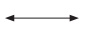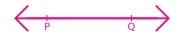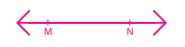Home | | Maths 5th Std | Recall

# Recall

Point, line, line segment and Ray, Types of Angles

UNIT−1

GEOMETRYRecall:

Point, line, line segment and Ray:

point

A point is an exact position on a plane surface

lineA line is a set of points in a straight path that extends in opposite directions without ending.

line segmentA line segment is a part of a line between two end points.

RayA ray is a part of a line that has one end point and extends in one direction without ending.

Types of AnglesTry this

1. Tick () the correct alternative

i.The shortest distance between the points C and D is shown by the segment CD / the curve CD

ii.line PQ and line QP represent different lines / the same line

iii.point C lies on the ray AB/ray BD

iv.Segment MN has infinite / finite length

v.Ray RT is a part / is not a part of the line TR

2. Write the type of the angle(i) Right angle

(ii) Acute angle

(iii) Straight angle

(iv) Obtuse angle

Tags : Geometry | Term 2 Chapter 1 | 5th Maths , 5th Maths : Term 2 Unit 1 : Geometry
Study Material, Lecturing Notes, Assignment, Reference, Wiki description explanation, brief detail
5th Maths : Term 2 Unit 1 : Geometry : Recall | Geometry | Term 2 Chapter 1 | 5th Maths